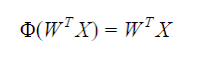## 2017年2月14日 星期二

<圖二>Rosenblatt感知器(Perceptron)

### 數學模型

"單位階梯函數"(unit step function)。如下：

下圖說明了，淨輸入z是如何被啟動函數(左圖)壓縮成二元輸出(-1 or 1)，而右圖則是說明淨輸入如何被二元分成兩類。而啟動函數Φ(z)實質的意義就是會把淨輸入的值轉換對應到該啟動函數的線上。例如Rosenblatt感知器使用的單位階梯函數，會把淨輸入z直接分成整數值1或-1。
而如果用sigmoid 函數，淨輸入的值Z會被轉成最大靠近1.0最小靠近0.0。

### 以遞減梯度法最小化成本函數

監督式學習演算法的一個關鍵要素是定義"目標函數"，這個函數在處裡過程中被最佳化

j為某一w加權index。

<圖四>成本函數J(w)相對於加權wj的偏導數推導，底下E(w)即是J(w)

import pandas as pd

<完整範例程式：>

```import pandas as pd
import matplotlib.pyplot as plt
import numpy as np
from matplotlib.colors import ListedColormap

#劃出分布圖及分類結果+
def plot_decision_regions(X, y, classifier, resolution=0.02):

# setup marker generator and color map
markers = ('s', 'x', 'o', '^', 'v')
colors = ('red', 'green', 'lightgreen', 'gray', 'cyan')
#np.unique =>Find the unique elements of an array
cmap = ListedColormap(colors[:len(np.unique(y))])
#len(np.unique(y))=2
# plot the decision surface
x1_min, x1_max = X[:, 0].min() - 1, X[:, 0].max() + 1 #feature 1
x2_min, x2_max = X[:, 1].min() - 1, X[:, 1].max() + 1 #feature 2

xx1, xx2 = np.meshgrid(np.arange(x1_min, x1_max, resolution),
np.arange(x2_min, x2_max, resolution))
Z = classifier.predict(np.array([xx1.ravel(), xx2.ravel()]).T)
Z = Z.reshape(xx1.shape)

plt.contourf(xx1, xx2, Z, alpha=0.4, cmap=cmap)
plt.xlim(xx1.min(), xx1.max())
plt.ylim(xx2.min(), xx2.max())

# plot class samples
for idx, cl in enumerate(np.unique(y)):
#idx=0,1  ;cl=-1,1
print("X[y == cl, 0]=",X[y == cl, 0])
print("X[y == cl, 1]=",X[y == cl, 1])
plt.scatter(x=X[y == cl, 0], y=X[y == cl, 1],
alpha=0.8, c=cmap(idx),
marker=markers[idx], label=cl)
#劃出分布圖及分類結果-

Parameters
------------
eta : float
Learning rate (between 0.0 and 1.0)
n_iter : int
Passes over the training dataset.

Attributes
-----------
w_ : 1d-array
Weights after fitting.
cost_ : list
Sum-of-squares cost function value in each epoch.

"""
def __init__(self, eta=0.01, n_iter=50):
self.eta = eta
self.n_iter = n_iter

def fit(self, X, y):
""" Fit training data.

Parameters
----------
X : {array-like}, shape = [n_samples, n_features]
Training vectors, where n_samples is the number of samples and
n_features is the number of features.
y : array-like, shape = [n_samples]
Target values.

Returns
-------
self : object

"""
self.w_ = np.zeros(1 + X.shape)
self.cost_ = []

for i in range(self.n_iter):
net_input = self.net_input(X)
# Please note that the "activation" method has no effect
# in the code since it is simply an identity function. We
# could write `output = self.net_input(X)` directly instead.
# The purpose of the activation is more conceptual, i.e.,
# in the case of logistic regression, we could change it to
# a sigmoid function to implement a logistic regression classifier.
output = self.activation(X)
errors = (y - output)
self.w_[1:] += self.eta * X.T.dot(errors)
self.w_ += self.eta * errors.sum()
cost = (errors**2).sum() / 2.0
self.cost_.append(cost)
return self

def net_input(self, X):
"""Calculate net input"""
return np.dot(X, self.w_[1:]) + self.w_

def activation(self, X):
"""Compute linear activation"""
return self.net_input(X)

def predict(self, X):
"""Return class label after unit step"""
return np.where(self.activation(X) >= 0.0, 1, -1)

#使用pandas讀進iris.data' 格式為CSV+
#擷取出類別標籤
y = df.iloc[0:100, 4].values
#將類別標籤定義為-1及1兩類
y = np.where(y == 'Iris-setosa', -1, 1)
#擷取出花萼長sepal length and 及花瓣petal length
X = df.iloc[0:100, [0, 2]].values
#使用pandas讀進iris.data' 格式為CSV-
#標準化資料+
X_std = np.copy(X)
X_std[:, 0] = (X[:, 0] - X[:, 0].mean()) / X[:, 0].std()
X_std[:, 1] = (X[:, 1] - X[:, 1].mean()) / X[:, 1].std()
#標準化資料-

#畫出每一輪的成本下降圖
plt.subplot(211)
plt.xlabel('Epochs')
plt.ylabel('Sum-squared-error')
plt.tight_layout()

#畫出決策區域圖
plt.subplot(212)
plt.xlabel('sepal length [standardized]')
plt.ylabel('petal length [standardized]')
plt.legend(loc='upper left')
plt.tight_layout()
plt.show()

```

<參考資料>書名：Python機器學習，作者：Sebastian Raschka

<其他相關文章>